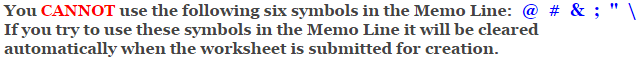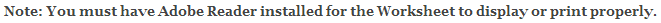# Fractions Worksheets

## Adding and Subtracting Three Fractions Worksheets

This fractions worksheet is great for testing children in adding and subtracting three fractions. The problems may be selected for five different degrees of difficulty.
The easiest will keep the denominators the same and the numerators between 1 and 9.
The hardest will keep the numerators between 1 and 20 and the common denominators under 120.
The answer worksheet will show the progression on how to solve the problems.
First find the common denominator and adjust the fractions.
The next step is to add the numerators.
Then check to see if we need to simplify or reduce the fraction, and finally check to see if it is an improper fraction if it is we convert it to a mixed fraction.

### Difficulty

Numerators 1 thru 9.
Denominators 1 thru 12.
Both fractions have the same denominator.

Numerators 1 thru 9.
Denominators 2, 3, 4, 5, & 10.

Numerators 1 thru 12.
Common denominators up to 30.

Numerators 1 thru 16.
Common denominators up to 60.

Numerators 1 thru 20.
Common denominators up to 120.

### Language for the Fractions Worksheet

 English German Albanian Spanish Swedish Italian French Turkish Polish Norwegian

### Memo Line for the Fractions Worksheet

You may enter a message or special instruction that will appear on the bottom left corner of the Fractions Worksheet.### Fractions Worksheet Answer Page

Now you are ready to create your Fractions Worksheet by pressing the Create Button.

 Recommended Videos

If You Experience Display Problems with Your Math WorksheetClick here for More Fractions WorksheetsMath-Aids.Com.  All rights reserved. Fractions Worksheets